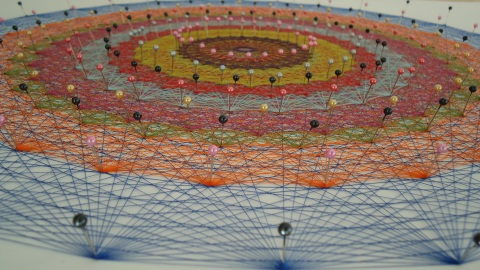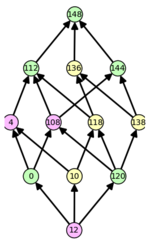# Research in Lie Algebra Cohomology

As a postdoc at UGA, I became involved in the VIGRE Algebra research group. This is a large research group including graduate students, postdocs, and senior faculty, working for several years on projects in representation theory of algebraic groups. The focus is on Lie algebra cohomology, and thus there are a number of connections with tools from algebraic topology. Moreover, there are parts of the work that can be reduced to finite (but non-trivial) Weyl group calculations, and thus to computer programs—one does not need to have a strong background in algebraic groups for this aspect of the project.

## Low-Degree Lie Algebra Cohomology ¶

This project is joint with the UGA VIGRE Algebra Group.
Slides summarizing degree-one and degree-two results (September 2011).

### First cohomology for finite groups of Lie type: simple modules with small dominant weights

arXiv 1010.1203 (2010): [abs | pdf]

#### Abstract:

Let $$k$$ be an algebraically closed field of characteristic $$p > 0$$, and let $$G$$ be a simple, simply connected algebraic group defined over $$\mathbb{F}_p$$. Given $$r \geq 1$$, set $$q=p^r$$, and let $$G(\mathbb{F}_q)$$ be the corresponding finite Chevalley group. In this paper we investigate the structure of the first cohomology group $$H^1(G(\mathbb{F}_q),L(\lambda))$$ where $$L(\lambda)$$ is the simple $$G$$-module of highest weight $$\lambda$$. Under certain very mild conditions on $$p$$ and $$q$$, we are able to completely describe the first cohomology group when $$\lambda$$ is less than or equal to a fundamental dominant weight. In particular, in the cases we consider, we show that the first cohomology group has dimension at most one. Our calculations significantly extend, and provide new proofs for, earlier results of Cline, Parshall, Scott, and Jones, who considered the special case when $$\lambda$$ is a minimal nonzero dominant weight.

### Second Cohomology for Finite Groups of Lie Type

arXiv 1110.0228 (2011): [abs | pdf]

#### Abstract:

Let $$G$$ be a simple, simply-connected algebraic group defined over $$\mathbb{F}_p$$. Given a power $$q = p^r$$ of $$p$$, let $$G(\mathbb{F}_q) \subset G$$ be the subgroup of $$\mathbb{F}_q$$-rational points. Let $$L(\lambda)$$ be the simple rational $$G$$-module of highest weight $$\lambda$$. In this paper we establish sufficient criteria for the restriction map in second cohomology $$H^2(G,L(\lambda)) \rightarrow H^2(G(\mathbb{F}_q),L(\lambda))$$ to be an isomorphism. In particular, the restriction map is an isomorphism under very mild conditions on $$p$$ and $$q$$ provided $$\lambda$$ is less than or equal to a fundamental dominant weight. Even when the restriction map is not an isomorphism, we are often able to describe $$H^2(G(\mathbb{F}_q),L(\lambda))$$ in terms of rational cohomology for $$G$$. We apply our techniques to compute $$H^2(G(\mathbb{F}_q),L(\lambda))$$ in a wide range of cases, and obtain new examples of nonzero second cohomology for finite groups of Lie type.

## Weyl Group Calculations for Linkage of Dominant Weights ¶

Two dominant weights, $$\lambda$$ and $$\tau$$, of a reductive algebraic group are linked over $$p > 0$$ if they are in the same orbit of the affine Weyl group, $$W_p$$. The Linkage Principle gives a (non-)linkage condition for vanishing of certain $$Ext^1$$ groups between highest-weight modules, and thus an avenue for approaching calculations of Lie algebra cohomology by computer.Construction showing a two-dimensional projection of the 248 8-dimensional root vectors of E8. The image comes from the series Hilorama de E8.

We developed a package available here for the computational algebra program GAP. This package determines whether given dominant weights are linked with each other at given primes; a future version will be extended to compute additional linkage data (e.g. strong linkage, very strong linkage), with potential applications to calculations of translation functors and other fine structure of modules over an algebraic group.

## Composition factors of Weyl modules ¶Weyl module V(omega_148) for C_2185 at the prime 3.

Adamovich and Kleshchev-Sheth give combinatorial algorithms for determining the composition factors of certain algebraic groups and the symmetric groups. As part of the project on low-degree cohomology, we developed Sage code to implement these algorithms and draw the resulting posets of composition factors.

If you're interested in using it, the source code is completely documented (over 400 doctests) and available under the GPL. And if you have further questions or comments, I would love to hear from you! To use the code, just download the .py Sage source file, and load it into your Sage session. The .patch file is an alternative format; just ignore it if you don't know what it is.